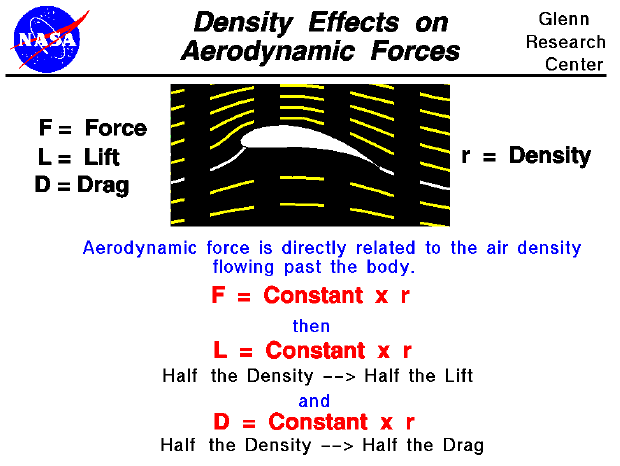Lift is created by deflecting a moving fluid (liquid or gas), and drag is generated on a body in a wide variety of ways. From Newton's second law of motion, the aerodynamic forces on the body (lift and drag) are directly related to the change in momentum of the fluid with time. The fluid momentum is equal to the mass times the velocity of the fluid. Since the fluid is moving, defining the mass gets a little tricky. If the mass of fluid were brought to a halt, it would occupy some volume in space; and we could define its density to be the mass divided by the volume. With a little math which is described on the fluid momentum page, we can show that the aerodynamic forces are directly proportional to the density of the fluid that flows by the airfoil.

Lift and drag depend linearly on the density of the fluid. Halving the density halves the lift, halving the density halves the drag. The fluid density depends on the type of fluid and the depth of the fluid. In the atmosphere, air density decreases as altitude increases. This explains why airplanes have a flight ceiling, an altitude above which it cannot fly. As an airplane ascends, a point is eventually reached where there just isn't enough air mass to generate enough lift to overcome the airplane's weight. The relation between altitude and density is a fairly complex exponential that has been determined by measurements in the atmosphere.

Let's investigate the dependence of lift on density using a Java simulator.

As an experiment, set the altitude to 5300 feet and note the value of the density and the amount of lift. Now change the altitude to 26,500 feet. What is the new value of the density? What is the value of the lift? How do these compare to the previous measurement? Notice that the altitude did not double. Now take this airplane to Mars. How does the density and lift compare to Earth at the same altitude? Move the altitude up to 37000 feet. How does the density and lift compare to the original measurements on Earth?

You can further investigate the effect of density and the other factors affecting lift by using the FoilSim II Java Applet. You can also download your own copy of FoilSim to play with for free.Guided Tours

Beginner's Guide to Aerodynamics
Beginner's Guide to Propulsion
Beginner's Guide to Model Rockets
Beginner's Guide to Kites
Beginner's Guide to Aeronautics